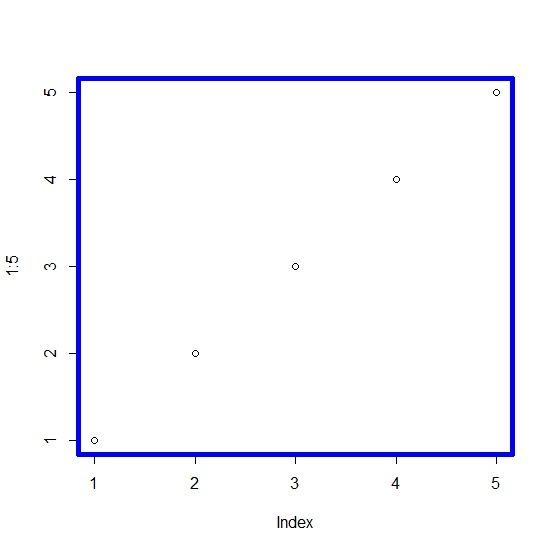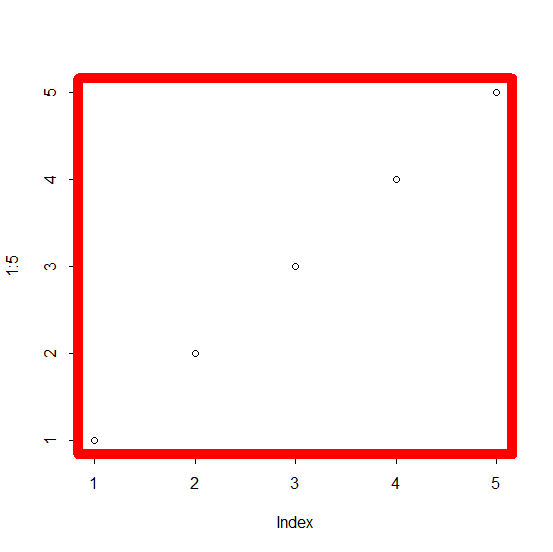# How to create a colored box for base R plot?

To create a colored box for base R plot, we can use box function with col argument where we can pass the color other than black because black is the default color.

We first need to create the plot using plot function and then box function will be used as shown in the below given example.

## Example

To create a colored box for base R plot, use the following code −

plot(1:5)
box(lwd=5,col="blue")

## Output

If you execute the above given code, it generates the following output −To create a colored box for base R plot, use the following code −

plot(1:5)
box(lwd=10,col="red")

## Output

If you execute the above given code, it generates the following output −